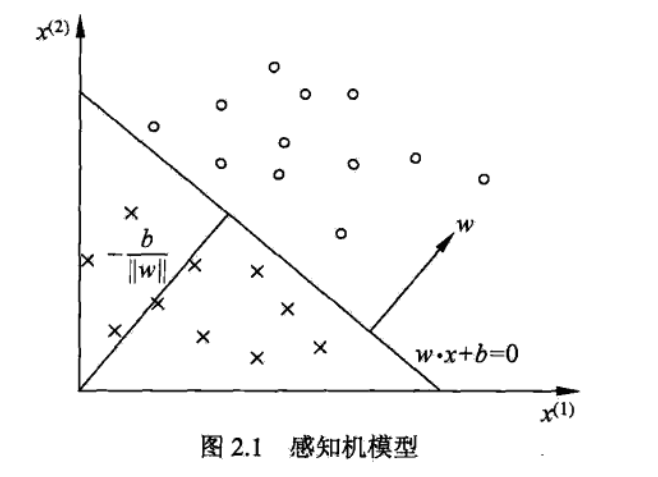1： 感知机模型

2: 感知机学习策略

• 2.1 数据集的线性可分
• 2.2 感知机学习策略

3： 感知机学习算法

• 3.1 感知机学习算法的原始形式
• 3.2 算法的收敛性
• 3.3 感知机python实现
• 3.4 感知机学习算法的对偶形式

4：参考文献

## 1： 感知机模型## 2: 感知机学习策略

2.1 数据集的线性可分2.2 感知机学习策略## 3： 感知机学习算法

3.1 感知机学习算法的原始形式1. 选取初值2. 在训练集中选取数据3. 如果4. 转至2，直至训练集中没有误分类点。

3.2 算法的收敛性

（1）存在满足条件的超平面将训练数据集完整正确分开；并存在，对所有（2）令，则感知机算法在训练集上的误分类次数满足不等式：3.3 感知机python实现

import pandas as pd
import numpy as np
import cv2
import time
from sklearn.cross_validation import train_test_split
from sklearn.metrics import accuracy_score

class Perceptron(object):
def __init__(self):
self.learning_step = 0.00001
self.max_iteration = 5000

def predict_(self, x):
wx = sum([self.w[j] * x[j] for j in xrange(len(self.w))])
return int(wx > 0)

def train(self, features, labels):
self.w = [0.0] * (len(features) + 1)  #权重+偏置
correct_count = 0
time = 0

while time < self.max_iteration:
index = np.random.randint(0, len(labels) - 1) #随机找个样本
x = list(features[index])
x.append(1.0)  #偏置
y = 2 * labels[index] - 1
wx = sum([self.w[j] * x[j] for j in range(len(self.w))])

if wx * y > 0:
correct_count += 1
if correct_count > self.max_iteration:
break
continue

#错误就更新参数
for i in range(len(self.w)):
self.w[i] += self.learning_step * (y * x[i])

def predict(self,features):
labels = []
for feature in features:
x = list(feature)
x.append(1)
labels.append(self.predict_(x))
return labels

if __name__ == '__main__':

time_1 = time.time()
data = raw_data.values

imgs = data[0::, 1::]
labels = data[::, 0]

# 选取 2/3 数据作为训练集， 1/3 数据作为测试集
train_features, test_features, train_labels, test_labels = train_test_split(
imgs, labels, test_size=0.33, random_state=23323)

time_2 = time.time()
print('read data cost ', time_2 - time_1, ' second', '\n')

print('Start training')
p = Perceptron()
p.train(train_features, train_labels)

time_3 = time.time()
print('training cost ', time_3 - time_2, ' second', '\n')

print('Start predicting')
test_predict = p.predict(test_features)
time_4 = time.time()
print('predicting cost ', time_4 - time_3, ' second', '\n')

score = accuracy_score(test_labels, test_predict)
print("The accruacy socre is ", score)


3.4 感机学习算法的对偶形式1.2. 在训练集中选取数据3. 如果4. 转至2，直至训练集中没有误分类点。